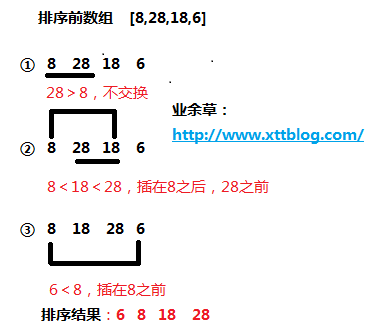# 排序算法之直接插入排序原理与实战

JAVA 2702浏览```public class InsertSort{
public void insertSort(int[] array){
for(int i=1;i<array.length;i++){ //第0位独自作为有序数列，从第1位开始向后遍历
if(array[i]<array[i-1]){ //0~i-1位为有序，若第i位小于i-1位，继续寻位并插入，否则认为0~i位也是有序的，忽略此次循环，相当于continue
int temp=array[i];//保存第i位的值
int k = i - 1;
for(int j=k;j>=0 && temp<array[j];j--){ //从第i-1位向前遍历并移位，直至找到小于第i位值停止
array[j+1]=array[j];
k--;
}
array[k+1]=temp;//插入第i位的值
}
}
}
public static void printArray(int[] array) {
for (int i = 0; i < array.length; i++) {
System.out.print(array[i]);
}
}
}```